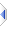# log

 Definition: atom res = log(atom x) -- or -- atom res = log10(atom x) -- or -- atom res = log2(atom x) Description: Return the natural or base 10/2 logarithm of x. Comments: This function may be applied to an atom or sq_log[10/2]() to all elements of a sequence. The rules for sequence operations apply. Note that log is only defined for positive numbers. Your program will abort with a message if you try to take the log of a negative number or zero. log10() is a simple wrapper to log(), multiplied by (1/log(10)). Likewise log2(). log() is directly supported by the floating point hardware and as such, without any wrapper/multiplication, may prove noticeably faster, and perhaps slightly more accurate. The builtin function exp(atom x) is the inverse of log(), and is implemented simply as return power(E,x). There is no similar builtin function for the inverse of log10(); you are expected to use power(10,x) directly, likewise log2(). Example: ```a = log(100) -- a is 4.60517 b = log10(100) -- b is 2.0 (approx) ``` Implementation: log(): via :%opLog in builtins\VM\pTrig.e (an autoinclude) log10() and log2(): see builtins\log10.e (an autoinclude) for details of the actual implementation. See Also: sin, cos, tan, sqrt, power